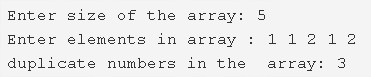# C Program Count Number of Duplicate Elements in An Array | C Programs

C program to count the total number of duplicate elements in an array – In this article, we will discuss the numerous methods to count the total number of duplicate elements in an array in C programming.

Suitable examples and sample programs have also been added so that you can understand the whole thing very clearly. The compiler has also been added with which you can execute it yourself.

The means used in this specific piece are as follows:

• Using Standard Method
• Using Function

An array, as you all know, is a general order or sequence of elements set in any possible manner. Arrays are specifically used to arrange the elements and the storage of those are denoted by pointers.As you can see in the photo uploaded above, firstly, you need to enter the size of the array concerned.

In this case, the size of the array was finalised at 5.

Then, the elements in the array are added accordingly.

The elements added in this particular array are as follows: 1 1 2 1 2

So, it is clear that out of the 5 elements, 3 elements are duplicate.

Thus, 3 will be printed as the final result.

Hence, the means to count the total number of duplicate elements in an array in C programming are as follows:

## Using Standard Method

1. Read the entered array size and store the value into the variable n and count initialized to 0.

2) Read the entered elements and store the elements in the array a[] as scanf(“%d”,&a[i]) using for loop for(i=0;i<n;i++).

3) Find the duplicate elements in the array as

for i=0 to i<n

a) If a[i]!=-1 then compare each element with remaining elements of the array. If any element repeated more than one time then increase the count value by 1. Repeat until j<n, using for loop for(j=i+1;j<n;j++).

4) After all iterations of i, print the count value c which represents the number of duplicate elements in the array.

Output:

### Using Function

1. The main() function calls the count() function passing array a, the size of the array are arguments to the function.

2) The function count() finds how many elements repeated in the array and returns the c value to main().

for i=0 to i<n

a) If a[i]!=-1

Then compare a[i] with remaining elements using for loop from j=i+1 t0 j<n.

If any element is equal to a[i] then increase the count value by 1. Like this repeat until j<n.

3) After all iterations of i,the count() function returns the c value to main() function then main() prints the c value.

Output:
x

## C Program To Print Number Of Days In A Month | Java Tutoring

C program to input the month number and print the number of days in that ...